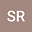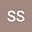Propagation of shock wave of the time fractional Gardner Burger equation in a multicomponent plasma using novel analytical method
•••• +1
• Subrata Roy,
• Sandip Saha,
• Santanu Raut,
• Apurba Das
Subrata Roy
Cooch Behar Panchanan Barma University

Corresponding Author:send2sroy94@gmail.com

Author ProfileSandip Saha
National Institute of Technology Silchar
Author ProfileSantanu Raut
Mathabhanga College
Author ProfileApurba Das
Alipurduar University
Author Profile## Abstract

The time-fractional Gardner Burger (TFGB) equation is an efficient model for studying nonlinear fluctuations of different types of wave profiles, such as the gravity solitary waves in the ocean, ion-acoustic wave (IAW) in a plasma environment, etc. Here, to build an example of the existence of the classical Gardner Burger (GB) equation, a multi-component plasma environment is considered and a classical GB equation is derived by employing reductive perturbation technique (RPT) from the basic governing equation. Further, the classical GB equation is converted into the TFGB equation by applying Agrawal’s approach, where the Riesz fractional derivative is adopted on the time-fractional term. A new approach using the improved Bernoulli sub-equation function method (IBSEFM) is carried out to solve the TFGB equation. Finally, some $2D$ and $3D$ graphs are plotted through which the physical structures of the solution are explored and the effect of the Burgers term and fractional order of the equation are determined.
25 Jun 2021Submitted to Mathematical Methods in the Applied Sciences
26 Jun 2021Submission Checks Completed
26 Jun 2021Assigned to Editor
03 Jul 2021Reviewer(s) Assigned
09 Nov 2022Editorial Decision: Revise Minor